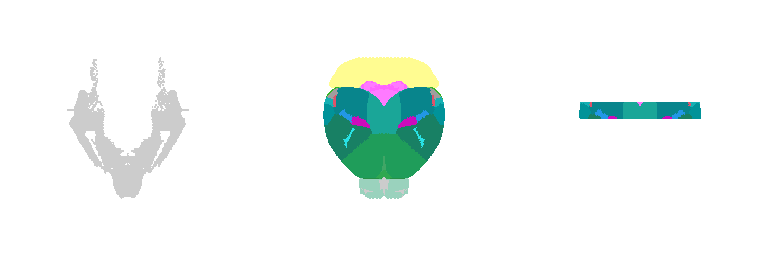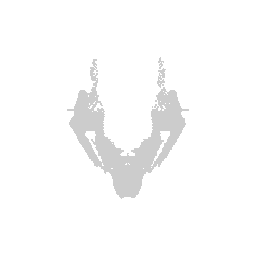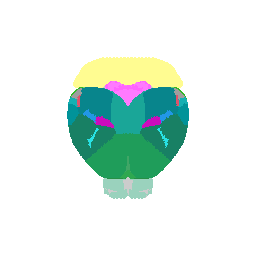# 3D animation of the brain in R

Nov 8, 2022## Backstory

Over the years, I have come to accept that if I don’t put code online, I will lose track of it and it will become lost in the ether. I wrote this code awhile ago for a presentation, then proceeded to forget where I saved the code, found the code again, and will now make a blog post for the code as a tutorial for future me. So in this blog post, I will explain to future me how to use data from the Allen Brain Atlas to animate a 3D brain using the `rgl` package in `R`.

## Getting the data

The Allen Brain Atlas offers downloads of 3D annotations of brain structure registered to their Mouse Common Coordinate Framework (CCF). Let’s get the brain structure annotations corresponding to the adult mouse brain CCF at 50um resolution.

``````## Download the 50um resolution 3D structural annotations
``````
``````## Registered S3 method overwritten by 'nat':
##   method             from
##   as.mesh3d.ashape3d rgl

## Warning in readBin(fc, what = dataTypes\$what[i], n = dataLength, size =
## dataTypes\$size[i], : 'signed = FALSE' is only valid for integers of sizes 1 and
## 2
``````
``````dim(annot)
``````
``````##  264 160 228
``````

Note that the data is in the form of a 264 by 160 by 228 matrix where each element is a structure ID. 0 corresponds to background.

``````## look at different small slices
annot[100:105,100:105,30]
``````
``````##      [,1] [,2] [,3] [,4] [,5] [,6]
## [1,]  120  120  120  120  120    0
## [2,]  163  120  120  120  120  120
## [3,]  163  163  120  120  120  120
## [4,]  163  163  163  120  120  120
## [5,]  163  163  163  163  120  120
## [6,]  163  163  163  163  163  120
``````
``````annot[100:105,100:105,50]
``````
``````##      [,1] [,2] [,3] [,4] [,5] [,6]
## [1,]  783  783  583  583  583  952
## [2,]  579  579  579  783  703  703
## [3,]  672  672  672  579  579  579
## [4,]  672  672  672  672  672  579
## [5,]  672  672  672  672  672  672
## [6,]  672  672  672  672  672  672
``````

We can obtain all the unique structure IDs and save them for future reference.

``````struc <- unique(as.numeric(annot))
``````
``````##    0 959  97 735 836 873
``````

The Allen Brain Atlas also has a mapping of all these structure IDs to acronyms as well as colors used in their interactive atlas viewer and other info. We cam parse through to get the acronym and color information corresponding to structure IDs info in a table format.

``````url <- 'http://api.brain-map.org/api/v2/structure_graph_download/1.json'
library(magrittr)
raw_json <- url %>%
httr::GET() %>%
httr::content()
json_tb <- tidyjson::json_structure(raw_json)
``````
``````## # A tbl_json: 6 x 10 tibble with a "JSON" attribute
##   ..JSON    document.id parent.id level index child.id seq    name  type  length
##   <chr>           <int> <chr>     <int> <int> <chr>    <list> <chr> <fct>  <int>
## 1 "true"              1 <NA>          0     1 1        <list> <NA>  logi…      1
## 2 "0"                 2 <NA>          0     1 1        <list> <NA>  numb…      1
## 3 "0"                 3 <NA>          0     1 1        <list> <NA>  numb…      1
## 4 "1"                 4 <NA>          0     1 1        <list> <NA>  numb…      1
## 5 "1"                 5 <NA>          0     1 1        <list> <NA>  numb…      1
## 6 "[{\"id\…           6 <NA>          0     1 1        <list> <NA>  array      1
``````
``````map <- do.call(rbind, lapply(1:nrow(json_tb), function(i) {
if(length(unlist(json_tb[i,]\$..JSON[])) > 6) {
c(
id = json_tb[i,]\$..JSON[]\$id,
atlas_id = json_tb[i,]\$..JSON[]\$atlas_id,
acronym = json_tb[i,]\$..JSON[]\$acronym,
col = paste0('#', json_tb[i,]\$..JSON[]\$color_hex_triplet)
)
}
}))
``````
``````## Warning in (function (..., deparse.level = 1) : number of columns of result is
## not a multiple of vector length (arg 23)
``````
``````map <- map[map[,1] != "#",]
``````
``````##      id          atlas_id acronym        col
## [1,] "997"       "-1"     "root"         "#FFFFFF"
## [2,] "8"         "0"      "grey"         "#BFDAE3"
## [3,] "1009"      "691"    "fiber tracts" "#CCCCCC"
## [4,] "73"        "716"    "VS"           "#AAAAAA"
## [5,] "1024"      "693"    "grv"          "#AAAAAA"
## [6,] "304325711" "retina" "#7F2E7E"      "304325711"
``````

For now, I will just focus on the mapping from structure IDs to colors.

``````allencol <- map[,4]
names(allencol) <- map[,1]
``````
``````##         997           8        1009          73        1024   304325711
##   "#FFFFFF"   "#BFDAE3"   "#CCCCCC"   "#AAAAAA"   "#AAAAAA" "304325711"
``````

## Plotting in 3D

In order to visualize these structures in 3D, I will get their 3D pixel coordinates at 50um resolution from the `annot` 3D matrix and also keep track of the structure’s corresponding colors using the `allencol` mapping vector we just made.

Let’s try visualizing one structure first. We will pick structure ID 1009, which corresponds to the fiber tracts.

``````teststruc <- "1009"
strucpos <- which(annot == teststruc, arr.ind=TRUE)
struccol <- rep(allencol[as.character(teststruc)], nrow(strucpos))
strucposall <- data.frame(strucpos, col=struccol)
``````
``````##   dim1 dim2 dim3     col
## 1  154  103   47 #CCCCCC
## 2  154  104   48 #CCCCCC
## 3  154  106   49 #CCCCCC
## 4  145   68   50 #CCCCCC
## 5  154  107   50 #CCCCCC
## 6  144   68   51 #CCCCCC
``````

We can visualize this structures as points in 3D using `rgl` and make some fun movies using the `movie3d()` and `spin3d()` functions in `rgl`.

``````library(rgl)
open3d()
view3d( theta = 90, phi = -90, fov = 0)
plot3d(strucpos[,1:3], col=struccol,
size=1, axes = FALSE,  xlab='', ylab='', zlab='',
aspect = dim(annot))
movie3d(spin3d(axis = c(1, 0, 0)), duration = 3, dir = getwd())
movie3d(spin3d(axis = c(0, 0, 1)), duration = 3, dir = getwd())
movie3d(spin3d(axis = c(0, 1, 0)), duration = 15, dir = getwd())
``````Now we can do loop through and do this for all the structures.

``````## ignore background struc
strucposall <- do.call(rbind, lapply(struc[-1], function(teststruc) {
strucpos <- which(annot == teststruc, arr.ind=TRUE)
struccol <- rep(allencol[as.character(teststruc)], nrow(strucpos))
data.frame(strucpos, col=struccol)
}))
``````
``````##   dim1 dim2 dim3     col
## 1  156   67   12 #019399
## 2  157   67   12 #019399
## 3  158   67   12 #019399
## 4  159   67   12 #019399
## 5  160   67   12 #019399
## 6  161   67   12 #019399
``````
``````open3d()
view3d( theta = 90, phi = -90, fov = 0)
plot3d(strucposall[,1:3], col=strucposall[,4],
size=1, axes = FALSE,  xlab='', ylab='', zlab='',
aspect = dim(annot))
movie3d(spin3d(axis = c(1, 0, 0)), duration = 3, dir = getwd())
movie3d(spin3d(axis = c(0, 0, 1)), duration = 3, dir = getwd())
movie3d(spin3d(axis = c(0, 1, 0)), duration = 15, dir = getwd())
``````We can even focus on a specific coronal slice cutting from 150 pixels to 180 pixels (multiple by 50um to mentally convert back to microns).

``````vi <- strucposall[,1] > 150 & strucposall[,1] < 180
view3d( theta = 90, phi = -90, fov = 0)
plot3d(strucposall[vi,1:3], col=strucposall[vi,4],
size=1, axes = FALSE,  xlab='', ylab='', zlab='',
aspect = dim(annot),
xlim = c(0, dim(annot)))
movie3d(spin3d(axis = c(1, 0, 0)), duration = 3, dir = getwd())
movie3d(spin3d(axis = c(0, 0, 1)), duration = 3, dir = getwd())
movie3d(spin3d(axis = c(0, 1, 0)), duration = 15, dir = getwd())
``````## Try it out for yourself

• Visualize a different brain structure.
• Visualize a different coronal slice.
• What if we use a higher resolution structural annotation to begin with?
• How do we integrate with meshes instead of discrete points?